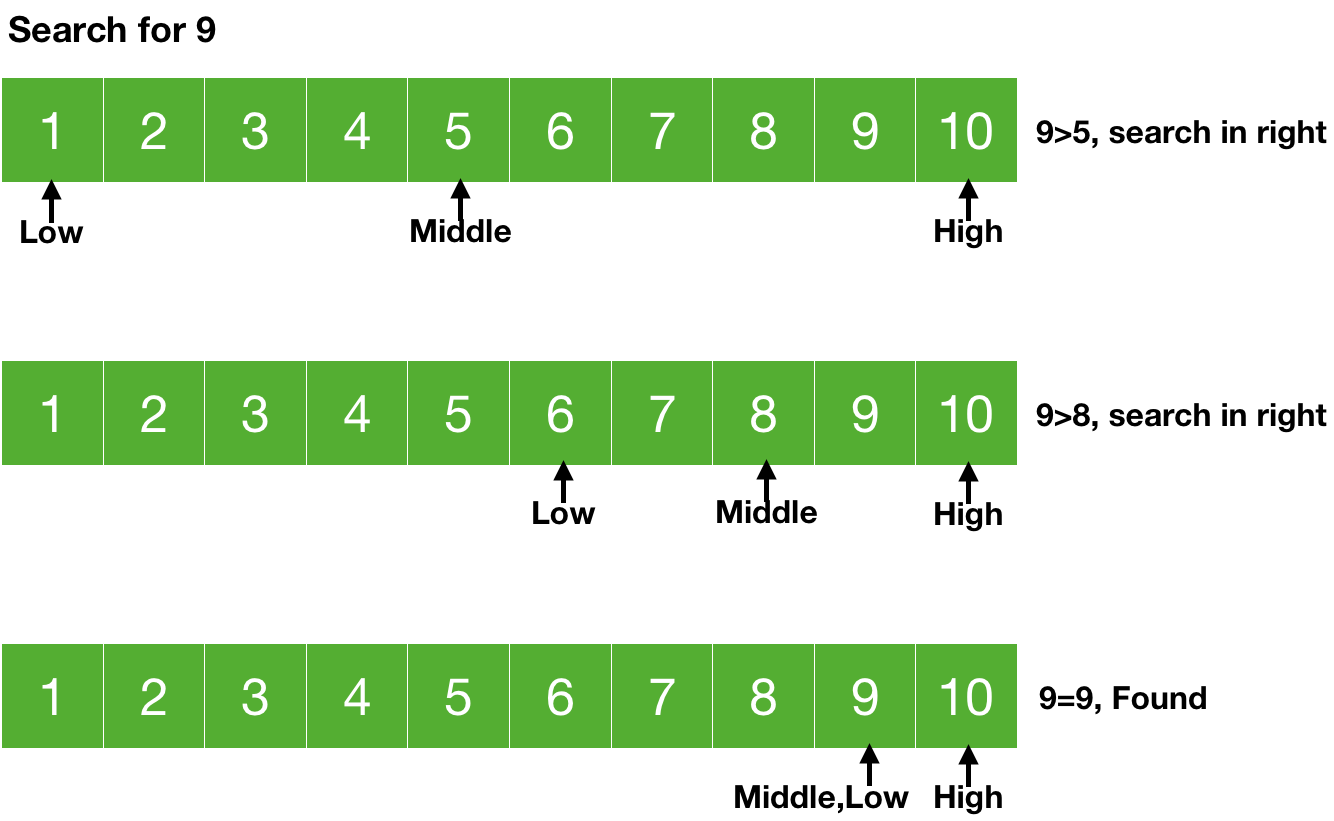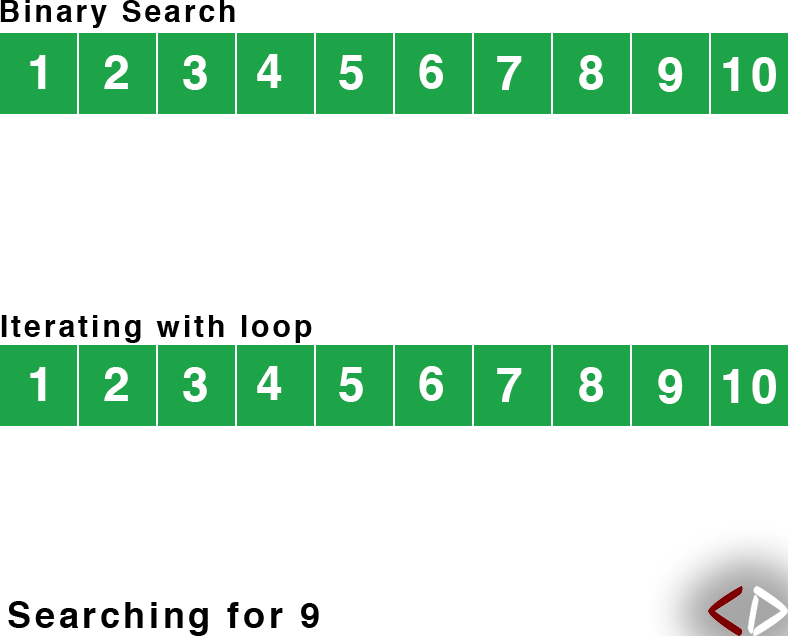# Binary Search

Nov. 19, 2018 5948

Suppose you have a sorted array and you want to search for an element in it, just iterating over each and every element will do the task but shouldn't we use something else, must faster if we know that our array is sorted? For example, let's just pick any element in the array and if the element to be searched is greater than the element we have picked then we would search for the element in the right side of the element (since the array is sorted, the larger element will be on the right side) and thus this will make our search much faster by eliminating many elements from the search. This is what binary search does. It first hits the middle element and then compares it with the element to be searched and if both are equal then we are done otherwise if it is greater, then we will search for the element in the right subarray (array from middle+1 element to last element) else, in the left subarray (array from the first element to the middle-1 element). In both the latter cases, we will repeat the process of binary search in the subarray and hit the middle element of the subarray. This is illustrated in the picture given below.You can see that in every repetition the size of the problem is becoming half and thus the binary search algorithm runs in O(logn) time.

Let's write the binary search function

binary_search(ar, low, high, x)
if (low<=high) // we can break the array further
// the middle element
middle = (low+high)/2

// the element to be found is at the middle
if (ar[middle] == x)
return middle

// element to be found is greater than the element
// at the middle.
// The element to be found should be in the right side

if (ar[middle]>x)
return binary_search(ar, low, middle-1, x)

// the element is neither at the middle nor int the
// right side of middle, so it should be in the left side

return binary_search(ar, middle+1, high, x)
return null


if (low<=high) - We can further break the array.

int middle = (low+high)/2 - Calculating the index of the middle element.

if (ar[middle] == x) – if the middle element is the element to be found then the search is completed and we will simply return the index of this element in the next line - return middle.

if (ar[middle]>x) – Element to be found is smaller than the middle element, we will search in the left subarray (from start to middle-1) - return binary_search(ar, low, middle-1, x).

If two of the above cases are not satisfied then the middle element is larger than the element to be found and we will search in the right subarray (from midddle+1 to the last element) - return binary_search(ar, middle+1, high, x).

If nothing is returned from the function and the search is complete then we didn't find the element and thus the element doesn't exist in the array and so we are returning -1 to indicate that the element doesn't exist.

### C

#include <stdio.h>

// funtion to implement binary search
int binary_search(int ar[], int low, int high, int x)
{
if (low <= high) // we can break the array further
{
// the middle element
int middle = (low+high)/2;

// the element to be found is at the middle
if (ar[middle] == x)
return middle;

/*
element to be found is greater than the element
at the middle.
The element to be found should be in the right side
*/
if (ar[middle] > x)
return binary_search(ar, low, middle-1, x);

/*
the element is neither at the midddle nor int the
right side of middle, so it should be in the left
side
*/
return binary_search(ar, middle+1, high, x);
}
return -1;
}

int main()
{
// sorted array
int a[] = {1, 2, 3, 4, 5, 6, 7, 8, 9, 10};
int found_index = binary_search(a, 0, 9, 2);
printf("Found at index: %d\n", found_index);
return 0;
}


### Python

# funtion to implement binary search
def binary_search(ar, low, high, x):
if (low<=high): # we can break the array further
# the middle element
middle = (low+high)//2

# the element to be found is at the middle
if (ar[middle] == x):
return middle

'''
element to be found is greater than the element
at the middle.
The element to be found should be in the right side
'''
if (ar[middle]>x):
return binary_search(ar, low, middle-1, x)

'''
the element is neither at the midddle nor int the
right side of middle, so it should be in the left
side
'''
return binary_search(ar, middle+1, high, x)
return -1

if __name__ == "__main__":
#sorted array
a = [1, 2, 3, 4, 5, 6, 7, 8, 9, 10]
found_index = binary_search(a, 0, 9, 2)
print("Found at index:",found_index)


### Java

class BinarySearch {

// funtion to implement binary search
static int binary_search(int ar[], int low, int high, int x)
{
if (low<=high) // we can break the array further
{
// the middle element
int middle = (low+high)/2;

// the element to be found is at the middle
if (ar[middle] == x)
return middle;

/*
element to be found is greater than the element
at the middle.
The element to be found should be in the right side
*/
if (ar[middle]>x)
return binary_search(ar, low, middle-1, x);

/*
the element is neither at the midddle nor int the
right side of middle, so it should be in the left
side
*/
return binary_search(ar, middle+1, high, x);
}
return -1;
}

public static void main (String[] args) {
// sorted array
int a[] = {1, 2, 3, 4, 5, 6, 7, 8, 9, 10};
int found_index = binary_search(a, 0, 9, 2);
System.out.println("Found at index: "+found_index);
}
}


Liked the post?
Developer and founder of CodesDope.
0 COMMENT